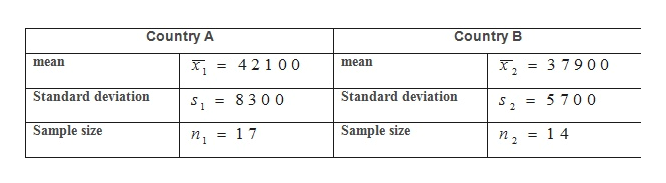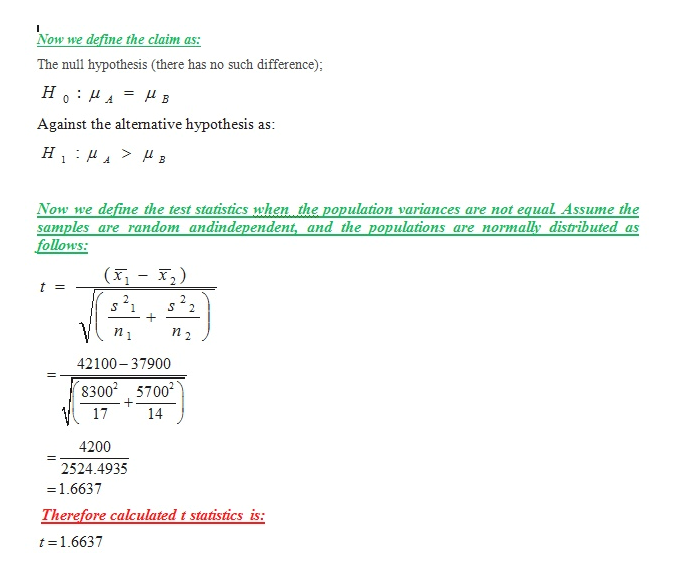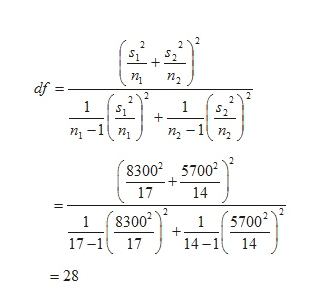A personnel director in a particular state claims that the mean annual income is the same in one of the​ state's counties​ (County A) as it is in another county​ (County B). In County​ A, a random sample of 17 residents has a mean annual income of \$42,100 and a standard deviation of \$8300.In County​ B, a random sample of 14 residents has a mean annual income of\$37,900 and a standard deviation of \$5700. At α=0.05,answer parts​ (a) through​ (e). Assume the population variances are not equal. Assume the samples are random and​ independent, and the populations are normally distributed.​(a) Identify the claim and stateUpper H 0H0andUpper H Subscript aHa. Which is the correct claim​ below? A.​"The mean annual incomes in Counties A and B are not​ equal." B.​"The mean annual incomes in Counties A and B are​ equal."This is the correct answer. C.​"The mean annual income in County A is greater than in County​ B." D.​"The mean annual income in County A is less than in County​ B."What are Upper H 0H0and Upper H Subscript aHa​? The null​ hypothesis,Upper H 0H0​,ismu 1 equals mu 2 .μ1=μ2. The alternative​ hypothesis,Upper H Subscript aHa​,ismu 1 not equals mu 2 .μ1≠μ2. Which hypothesis is the​ claim?  The alternative​ hypothesis, Upper H Subscript aHa The null​ hypothesis, Upper H 0H0Your answer is correct.​(b) Find the critical​ value(s) and identify the rejection​ region(s). Enter the critical​ value(s) below.

Question
A personnel director in a particular state claims that the mean annual income is the same in one of the​ state's counties​ (County A) as it is in another county​ (County B). In County​ A, a random sample of 17 residents has a mean annual income of \$42,100 and a standard deviation of \$8300.
In County​ B, a random sample of 14 residents has a mean annual income of
\$37,900 and a standard deviation of \$5700. At α=0.05,
answer parts​ (a) through​ (e). Assume the population variances are not equal. Assume the samples are random and​ independent, and the populations are normally distributed.
​(a) Identify the claim and state
Upper H 0H0
and
Upper H Subscript aHa.

Which is the correct claim​ below?

A.
​"The mean annual incomes in Counties A and B are not​ equal."

B.
​"The mean annual incomes in Counties A and B are​ equal."
This is the correct answer.

C.
​"The mean annual income in County A is greater than in County​ B."

D.
​"The mean annual income in County A is less than in County​ B."
What are Upper H 0H0
and Upper H Subscript aHa​?

The null​ hypothesis,
Upper H 0H0​,
is
mu 1 equals mu 2 .μ1=μ2.

The alternative​ hypothesis,
Upper H Subscript aHa​,
is
mu 1 not equals mu 2 .μ1≠μ2.

Which hypothesis is the​ claim?

The alternative​ hypothesis, Upper H Subscript aHa

The null​ hypothesis, Upper H 0H0
​(b) Find the critical​ value(s) and identify the rejection​ region(s).

Enter the critical​ value(s) below.
Step 1

According to the given question, in order to test that the annual income is the same in one of the​ state's counties​ (County A) as it is in another county​ (County B).

In County​ A, a random sample of 17 residents has a mean annual income of \$42,100 and a standard deviation of \$8300.
In County​ B, a random sample of 14 residents has a mean annual income of

\$37,900 and a standard deviation of \$5700.

Where we represent the entire data as:help_outlineImage TranscriptioncloseCountry A Country B mean ,3 7900 42100 mean 2 Standard deviation Standard deviation 8300 s,= 5700 S = Sample size Sample size 1 7 14 1 2 fullscreen
Step 2

We define the test hypothesis and the test statistics are as followsw;help_outlineImage TranscriptioncloseNow we define the claim as: The null hypothesis (there has no such difference); Но : иА A Against the altemative hypothesis as: н Now we define the test statistics when the population variances are not egual Assume the samples are random andindependent, and the populations are normally distributed as follows: (x ) 2 S 2 + n 42100-37900 83002 5700 17 14 4200 2524.4935 1.6637 Therefore calculated t statistics is: t 1.6637 fullscreen
Step 3

Where we can determine the d...help_outlineImage Transcriptionclosedf 1 1 1 n-1 n2-1n n 83002 57002 17 14 (5700 14-114 1 8300 17-1 17 1 =28 + + + fullscreen

Want to see the full answer?

See Solution

Want to see this answer and more?

Our solutions are written by experts, many with advanced degrees, and available 24/7

See Solution
Tagged in

Hypothesis Testing